Get inspired by the success stories of our students in IIT JAM MS, ISI  MStat, CMI MSc DS.  Learn More

# ISI MStat PSB 2009 Problem 5 | Finding the Distribution of a Random VariableThis is a very beautiful sample problem from ISI MStat PSB 2009 Problem 5 based on finding the distribution of a random variable . Let's give it a try !!

## Problem- ISI MStat PSB 2009 Problem 5

Suppose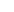andare continuous and strictly increasing distribution
functions. Let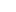have distribution functionand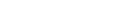(a) Find the distribution function of Y.
(b) Hence, or otherwise, show that the joint distribution function of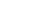denoted by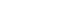is given by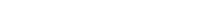.

### Prerequisites

Cumulative Distribution Function

Inverse of a function

Minimum of two function

## Solution :

(a) Let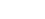be Cumulative distribution Function of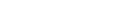Then ,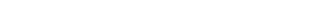=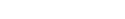[ taking G on both side, since G is Strictly in decreasing function the inequality doesn't change]
=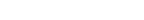[ taking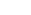on both side and since F is strictly increasing distribution function hence inverse exists and inequality doesn't change ]

=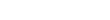[Since F is a distribution function of X ]
=G(y)

therefore Cumulative distribution Function ofis G .

(b) Let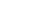be joint cdf of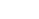then we have ,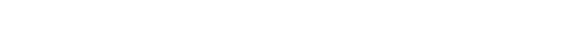=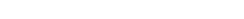[ Since if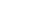with probability 1 then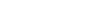with probability 1 as F is strictly increasing distribution function ]
=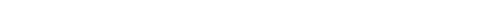=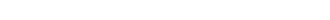[ Since F is CDF of X ]

Therefore , the joint distribution function ofdenoted byis given by## Food For Thought

Find the the distribution function ofwhere G is continuous and strictly decreasing function .

## Subscribe to Cheenta at Youtube

This is a very beautiful sample problem from ISI MStat PSB 2009 Problem 5 based on finding the distribution of a random variable . Let's give it a try !!

## Problem- ISI MStat PSB 2009 Problem 5

Supposeandare continuous and strictly increasing distribution
functions. Lethave distribution functionand(a) Find the distribution function of Y.
(b) Hence, or otherwise, show that the joint distribution function ofdenoted byis given by.

### Prerequisites

Cumulative Distribution Function

Inverse of a function

Minimum of two function

## Solution :

(a) Letbe Cumulative distribution Function ofThen ,=[ taking G on both side, since G is Strictly in decreasing function the inequality doesn't change]
=[ takingon both side and since F is strictly increasing distribution function hence inverse exists and inequality doesn't change ]

=[Since F is a distribution function of X ]
=G(y)

therefore Cumulative distribution Function ofis G .

(b) Letbe joint cdf ofthen we have ,=[ Since ifwith probability 1 thenwith probability 1 as F is strictly increasing distribution function ]
==[ Since F is CDF of X ]

Therefore , the joint distribution function ofdenoted byis given by## Food For Thought

Find the the distribution function ofwhere G is continuous and strictly decreasing function .

## Subscribe to Cheenta at Youtube

This site uses Akismet to reduce spam. Learn how your comment data is processed.

### Knowledge Partner